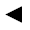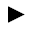﻿﻿﻿ math handbook calculator - Fractional Calculus Computer Algebra System software+++=﻿[back]

## 3.42. Pillow shape

[next]The pillow shape [ 8 ] is represented by the following equations.

 x = cos(u) 3-141 y = cos(v) 3-142 z = a sin(u) sin(v) 3-143

The constant a determines the appearance of the figure.

To represent the area, the two parameters u and v can assume the following values ​​(domain of definition), for example.

 u is an element from the set of numbers [0, pi] v is an element from the number set [-pi, pi]

Since the pillow shape is a closed figure, the definition range must be adhered to exactly, it cannot be changed with the plugin.